# Types of 2-D discrete data plots in MATLAB

• Difficulty Level : Easy
• Last Updated : 22 Sep, 2021

Any data or variable that is limited to having certain values is known as discrete data. Many examples of discrete data can be observed in real life such as:

• The output of a dice roll can take any whole number from 1 to 6.
• The marks obtained by any student in a test can range from 0 to 100.
• The number of children in a house.

When dealing with such data, we may require to plot graphs, histograms, or any other form of visual representation to analyze the data and achieve desired results.

MATLAB offers a wide variety of ways to plot discrete data. These include:

• Vertical or Horizontal Bar-graphs
• Pareto Charts
• Stem charts
• Scatter plots
• Stairs

Let us first take some sample 2-D data to work with while demonstrating these different types of plots.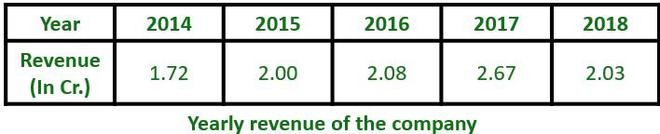The above data shows the yearly revenue of a company for the duration of 5 years. This data can be shown in any of the above-mentioned plots:

## Bar Graph:

This plot draws bars at positions specified by the array “Year” with the heights as specified in the array “Revenue”

Example:

## Matlab

 `% MATLAB code for Bar graph``% creating array for years``year = 2014:1:2018;`` ` `% creating array for revenue``revenue = [1.72 2.00 2.08 2.67 2.03];`` ` `% bar plot``bar(year,revenue)`` ` `% label for X-axis ``xlabel(``'Year'``);``   ` `% label for Y-axis ``ylabel(``'Revenue'``); `` ` `% title for plot``title(``'Yearly Revenue'``)`

Output: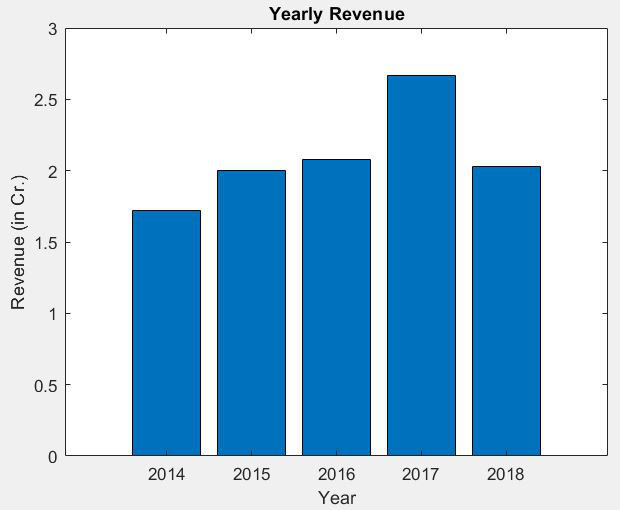## Horizontal Bar Graph:

This plot draws horizontal bars at positions specified by the array “Year” with the lengths as specified in the array “Revenue”.

Example:

## Matlab

 `% MATLAB code for horizontal bar graph``% creating array for years``year = 2014:1:2018;`` ` `% creating array for revenue``revenue = [1.72 2.00 2.08 2.67 2.03];`` ` `% horizontal bar plot``barh(year,revenue)`` ` `% label for X-axis ``xlabel(``'Revenue (in Cr.)'``);``   ` `% label for Y-axis ``ylabel(``'Year'``); `` ` `% title for plot``title(``'Yearly Revenue'``)`

Output: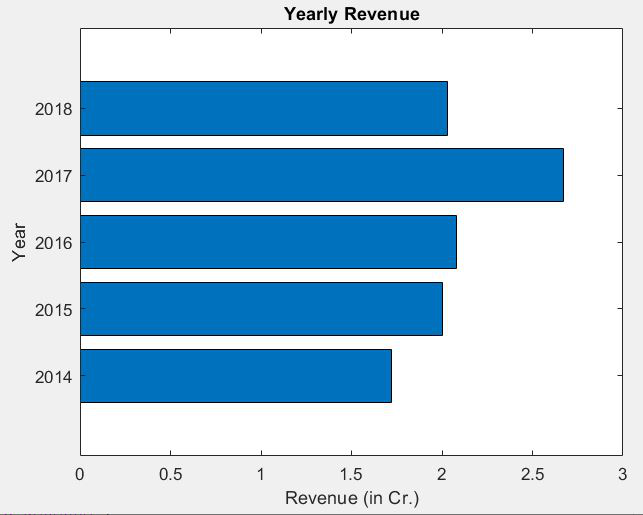## Pareto Charts:

This plot shows vertical bars corresponding to the values of the data in descending order of value. This also shows a curve made with the cumulative values above each bar. In addition to this, the right side of the graph has a percentage scale that shows how much percentage each bar contributes to the sum of all values.

Example:

## Matlab

 `% MATLAB code for Pareto Charts example``% creating array for years``year = 2014:1:2018;`` ` `% creating array for revenue``revenue = [1.72 2.00 2.08 2.67 2.03];`` ` `% pareto chart plot``pareto(revenue,year)`` ` `% label for X-axis ``xlabel(``'Year'``);``   ` `% label for Y-axis ``ylabel(``'Revenue (in Cr.)'``); `` ` `% title for plot``title(``'Yearly Revenue'``)`

Output: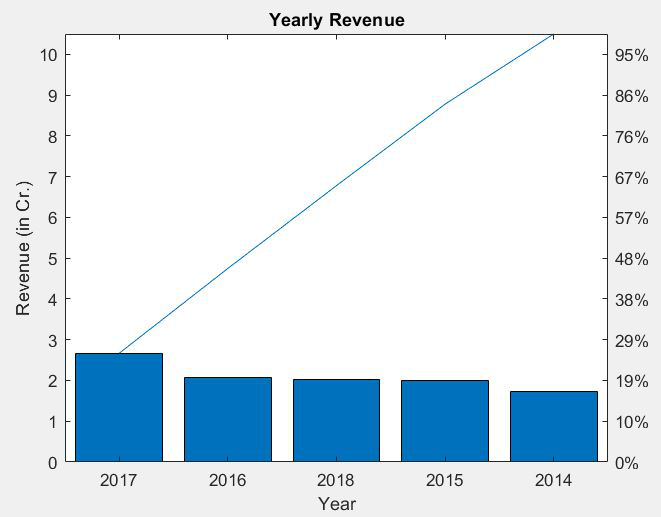Bar Graphs (both vertical and horizontal) and Pareto charts can be used to represent data such as marks of a student in different subjects, rainfall received in different months, and many other data sets.

## Stem Charts:

This plot shows a straight line with a bulb at the top (or bottom for negative values) corresponding to the values given in the data. The X-axis is scaled from the least to the highest value given. which may result in the first and last value being situated right at the border of the graph.

Example:

## Matlab

 `% MATLAB code for Stem Charts ``% creating array for years``year = 2014:1:2018;`` ` `% creating array for revenue``revenue = [1.72 2.00 2.08 2.67 2.03];`` ` `% stem chart plot``stem(year,revenue)`` ` `% label for X-axis ``xlabel(``'Year'``);``   ` `% label for Y-axis ``ylabel(``'Revenue (in Cr.)'``); `` ` `% title for plot``title(``'Yearly Revenue'``)`

Output: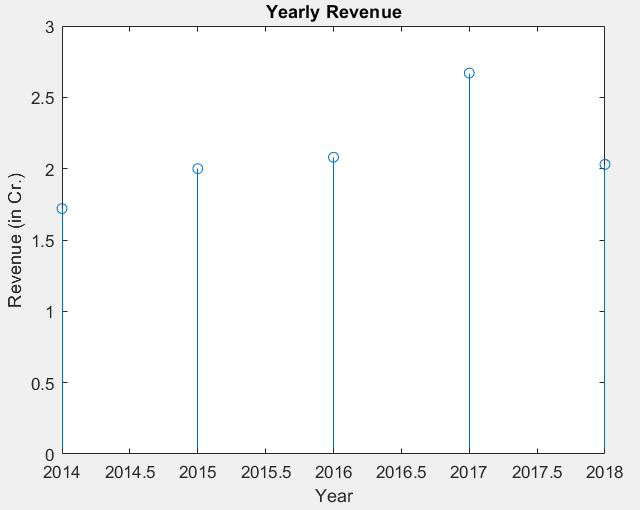## Scatter Plot:

This plot shows dots placed at the values given in the data. The Y-axis is scaled from the lowest to the highest value in the data. The X-axis is scaled similarly as in stem charts, from least to highest value.

Example:

## Matlab

 `% MATLAB code for Scatter Plot example``% creating array for years``year = 2014:1:2018;`` ` `% creating array for revenue``revenue = [1.72 2.00 2.08 2.67 2.03];`` ` `% scatter plot``scatter(year,revenue)`` ` `% label for X-axis ``xlabel(``'Year'``);``   ` `% label for Y-axis ``ylabel(``'Revenue (in Cr.)'``); `` ` `% title for plot``title(``'Yearly Revenue'``)`

Output: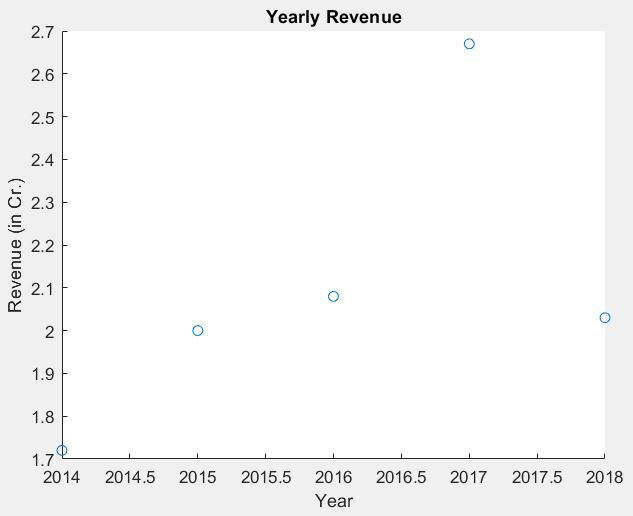## Stairstep Plot:

This plot shows a staircase-like structure with each step beginning at the next value given in the data. Similar to the scatter plot, X and Y axes scale from the lowest to the highest values given.

Example:

## Matlab

 `% MATLAB code for Stairstep Plot ``% creating array for years``year = 2014:1:2018;`` ` `% creating array for revenue``revenue = [1.72 2.00 2.08 2.67 2.03];`` ` `% stairstep plot``stairs(year,revenue)`` ` `% label for X-axis ``xlabel(``'Year'``);``   ` `% label for Y-axis ``ylabel(``'Revenue (in Cr.)'``); `` ` `% title for plot``title(``'Yearly Revenue'``)`

Output: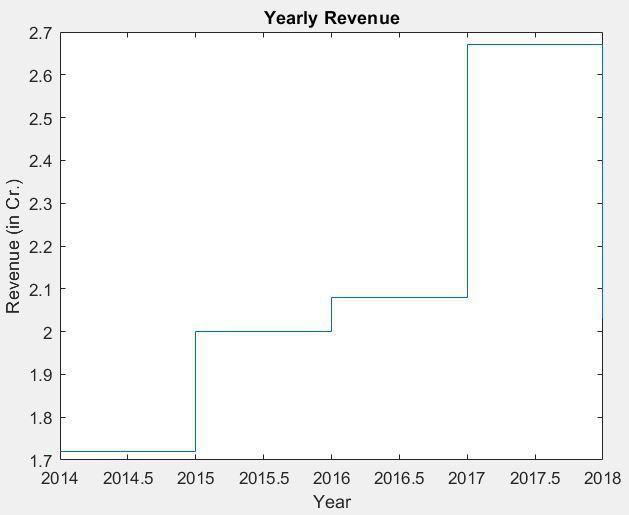Stem, Scatter, and Stairstep plots are ideally used when working with digital signals.

My Personal Notes arrow_drop_up